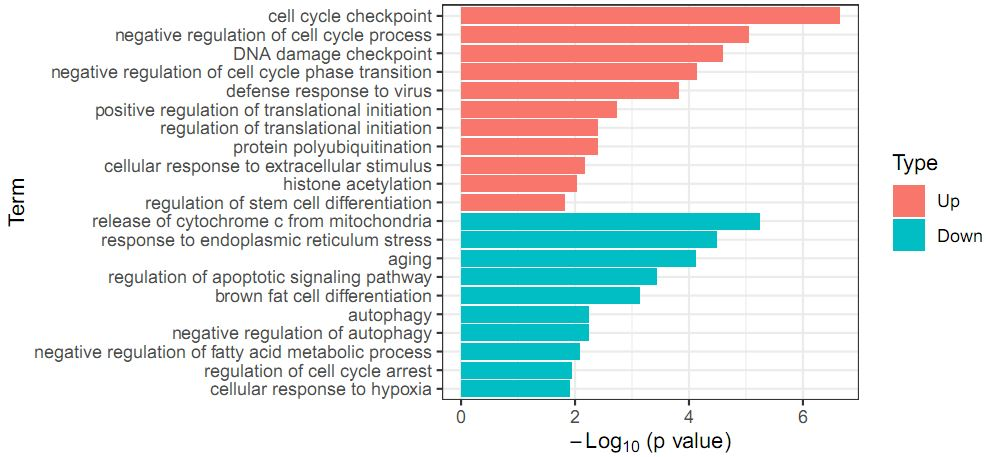# Run GO and KEGG enrichment#### Introduction

The following are the codes for GO and KEGG enrichment.

#### Code exmaple

``````library(clusterProfiler)
library(enrichplot)
library(tidyverse)
library(magrittr)
library(data.table)
library(org.Hs.eg.db)

setwd("/home/hxzk/project/XXX/KEGG/")

# Transform symbol to entrez id, here "gene name" is the column of gene symbol
xx <- unlist(as.list(org.Hs.egALIAS2EG))
map_dt <- data.table(Symbol = names(xx), ID = xx)
map_dt <- map_dt[!duplicated(Symbol),]
up_dt\$id <- map_dt[up_dt\$gene name,ID, on = "Symbol"]
down_dt\$id <- map_dt[down_dt\$gene name,ID, on = "Symbol"]

# Remove data without gene id
gene.ls <- list(Up = up_dt[!is.na(id),]\$id, Down = down_dt[!is.na(id)]\$id)

# Run KEGG and GO enrichment
compKEGG <- compareCluster(geneCluster   = gene.ls,
fun           = "enrichKEGG",
pvalueCutoff  = 0.05,
organism = "hsa") # or organism = "mmu" for mouse

compGO <- compareCluster(geneCluster   = gene.ls,
fun           = "enrichGO",
pvalueCutoff  = 0.05,
OrgDb = org.Hs.eg.db,
ont = 'BP') # or OrgDb = org.Mm.eg.db for mouse

up_eKEGG <- enrichKEGG(
gene = gene.ls[["Up"]],
pvalueCutoff = 0.05,
organism = 'hsa'
)
down_eKEGG <- enrichKEGG(
gene = gene.ls[["Down"]],
pvalueCutoff = 0.05,
organism = 'hsa'
)
up_eGO <- enrichGO(
gene          = gene.ls[["Up"]],
OrgDb         = org.Hs.eg.db,
ont           = "BP",
pvalueCutoff  = 0.05,
)
down_eGO <- enrichGO(
gene          = gene.ls[["Down"]],
OrgDb         = org.Hs.eg.db,
ont           = "BP",
pvalueCutoff  = 0.05,
)

get_GeneSymbol <- function(x){
x <- strsplit(x, split = "/", fixed = T)[]
symbol <- map_dt[ID %in% x, Symbol]
return(paste(symbol, collapse = "/"))
}

up_eKEGG@result\$Gene_symbol <- unlist(lapply(up_eKEGG@result\$geneID, get_GeneSymbol))
down_eKEGG@result\$Gene_symbol <- unlist(lapply(down_eKEGG@result\$geneID, get_GeneSymbol))

fwrite(up_eKEGG@result, file = "result/up_KEGG.txt", sep = "\t", col.names = T)
fwrite(down_eKEGG@result, file = "result/down_KEGG.txt", sep = "\t", col.names = T)
fwrite(up_eGO@result, file = "result/up_GO.txt", sep = "\t", col.names = T)
fwrite(down_eGO@result, file = "result/down_GO.txt", sep = "\t", col.names = T)
``````

Barplot codes for GO enrichment

``````library(data.table)
library(ggplot2)

setwd("/home/hxzk/project/Yuanyuan/KEGG/")

up_eGO_dt\$Type <- "Up"
down_eGO_dt\$Type <- "Down"

# Show top 10 up-regulated and down-regulated genes
dp <- rbind(up_eGO_dt[1:10,.(Term= Description, Pvalue=pvalue, Type)],
down_eGO_dt[1:10,.(Term=Description, Pvalue=pvalue, Type)])
dp\$Type <- factor(dp\$Type, levels = c("Up", "Down"))
dp <- dp[nrow(dp):1,]  # Up first
dp\$Term <- factor(dp\$Term, levels = dp\$Term)

p <- ggplot(dp, aes(x = Term, y = -log10(Pvalue))) +
geom_bar(aes(fill = Type), stat = "identity") + theme_bw()+
labs(x="Term", y=expression(-Log*" (p value)"))+ coord_flip()

ggsave(p, filename = "result/GO_enrichment.pdf", width = 7, height = 3.3)
``````

None

None